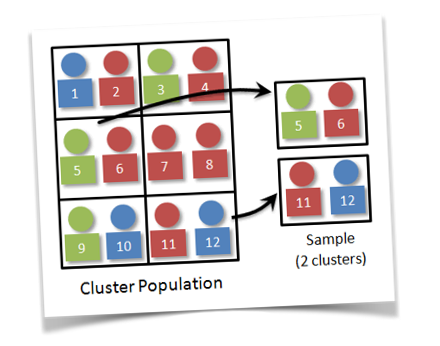# 4.2 Probability Sampling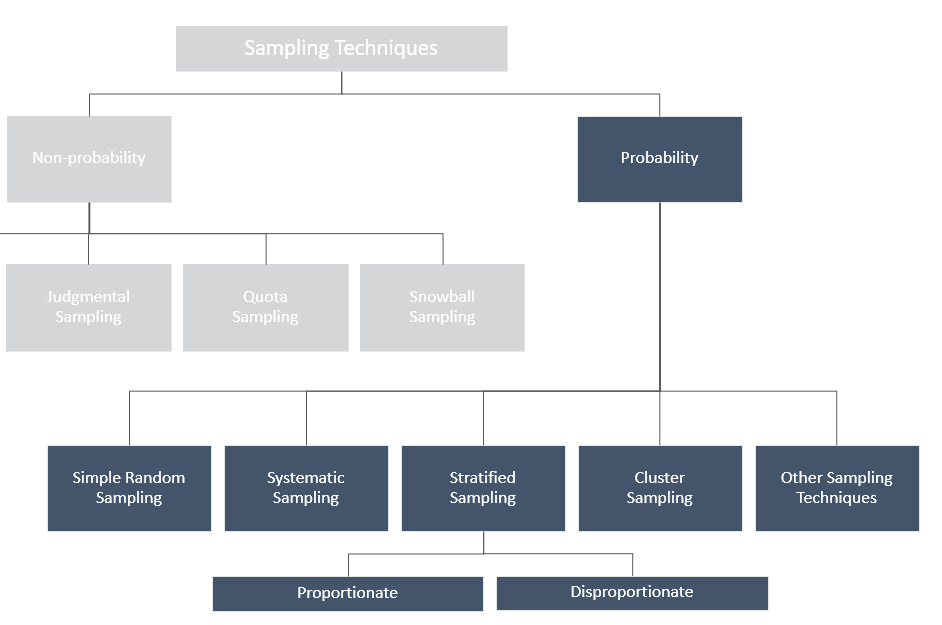### Simple Random Sampling & Systematic Sampling

Simple Random Sampling

• Each element in the population has a known and equal probability of selection
• Each possible sample of a given size (n) has a known probability of being the sample actually selected
• This implies that every element is selected independently of every other element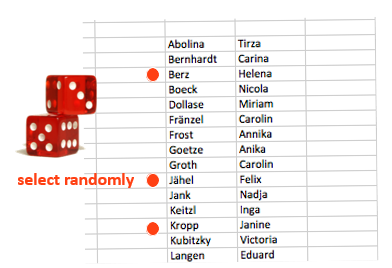Systematic Sampling

• The sample is chosen by selecting a random starting point and then picking every i-th element in succession from the sampling frame
• The sampling interval, i, is determined by dividing the population size N by the sample size n, i.e., i=N/n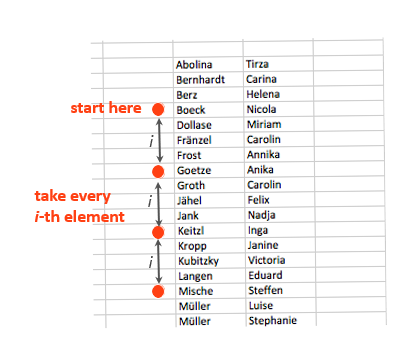### Stratified Sampling

Stratified sampling is obtained by separating the population into non-overlapping groups called strata and then obtaining a proportional simple random sample from each group. The individuals within each group should be similar in some way.

Good for:

• highlighting a specific subgroup within the population
• observing existing relationships between two or more subgroups
• representative sampling of even the smallest and most inaccessible subgroups in the population
• a higher statistical precision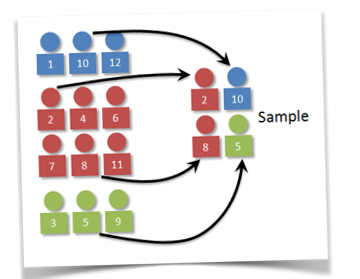Proportionate

 Stratum A B C Population Size 100 200 300 Sampling Fraction 1/2 1/2 1/2 Final Sample Size 50 100 150

Disproportionate

 Stratum A B C Population Size 100 200 300 Sampling Fraction 1/5 1/2 1/3 Final Sample Size 20 100 100

### Cluster Sampling

Cluster sampling the target population is first divided into mutually exclusive and collectively exhaustive subpopulations, or clusters. Than a random sample of clusters is selected, based on SRS.

Good for:

• covering large geographic areas
• reducing survey costs
• when constructing a complete list of population elements is difficult
• when the population concentrated in natural clusters (e.g., blocks, cities, schools, hospitals, boxes, etc.)

For each cluster, either all the elements are included in the sample (one-stage) or a sample of elements is drawn probabilistically (two-sage).